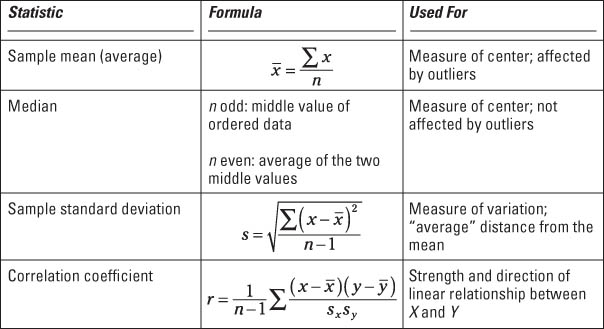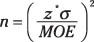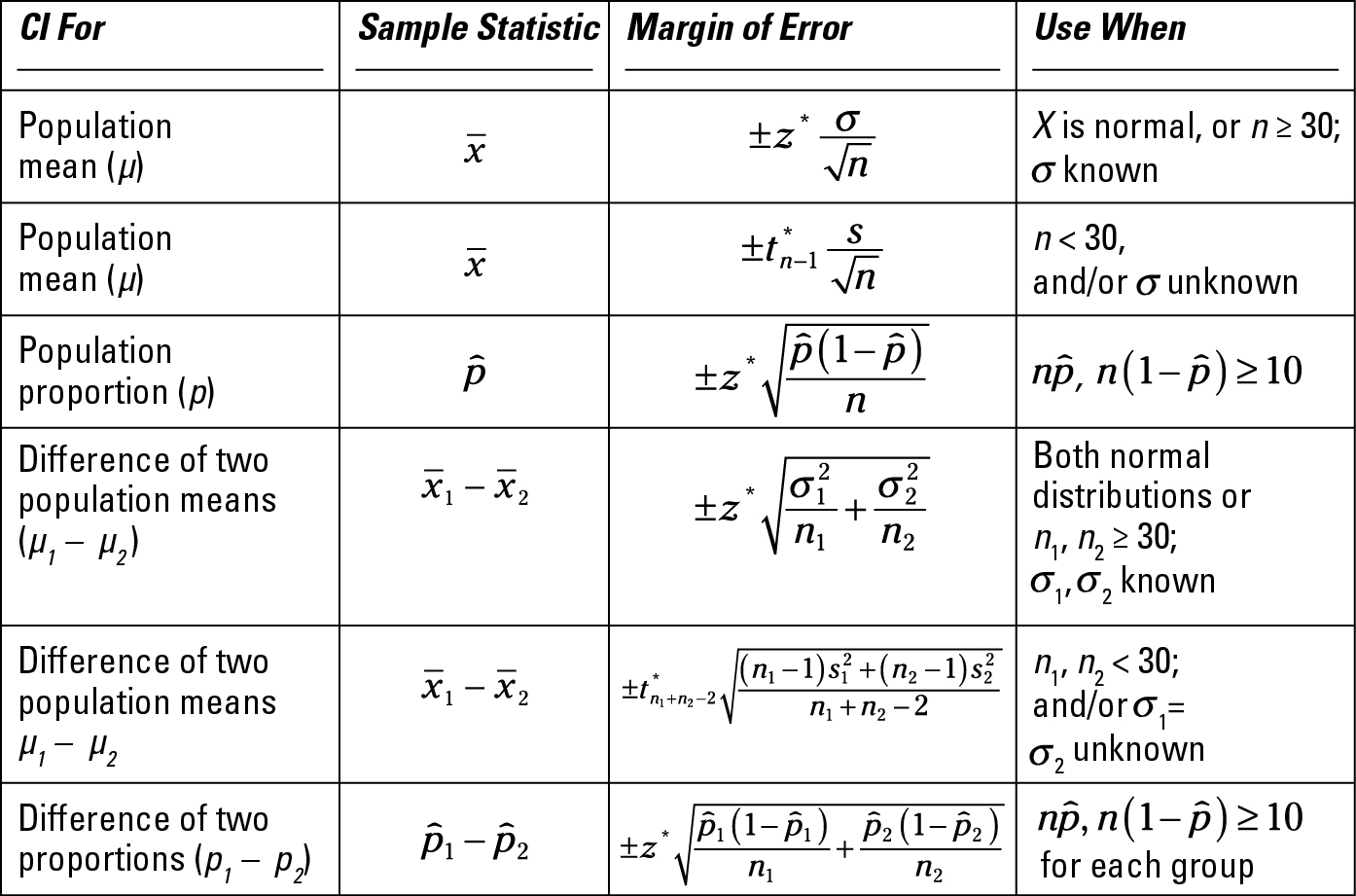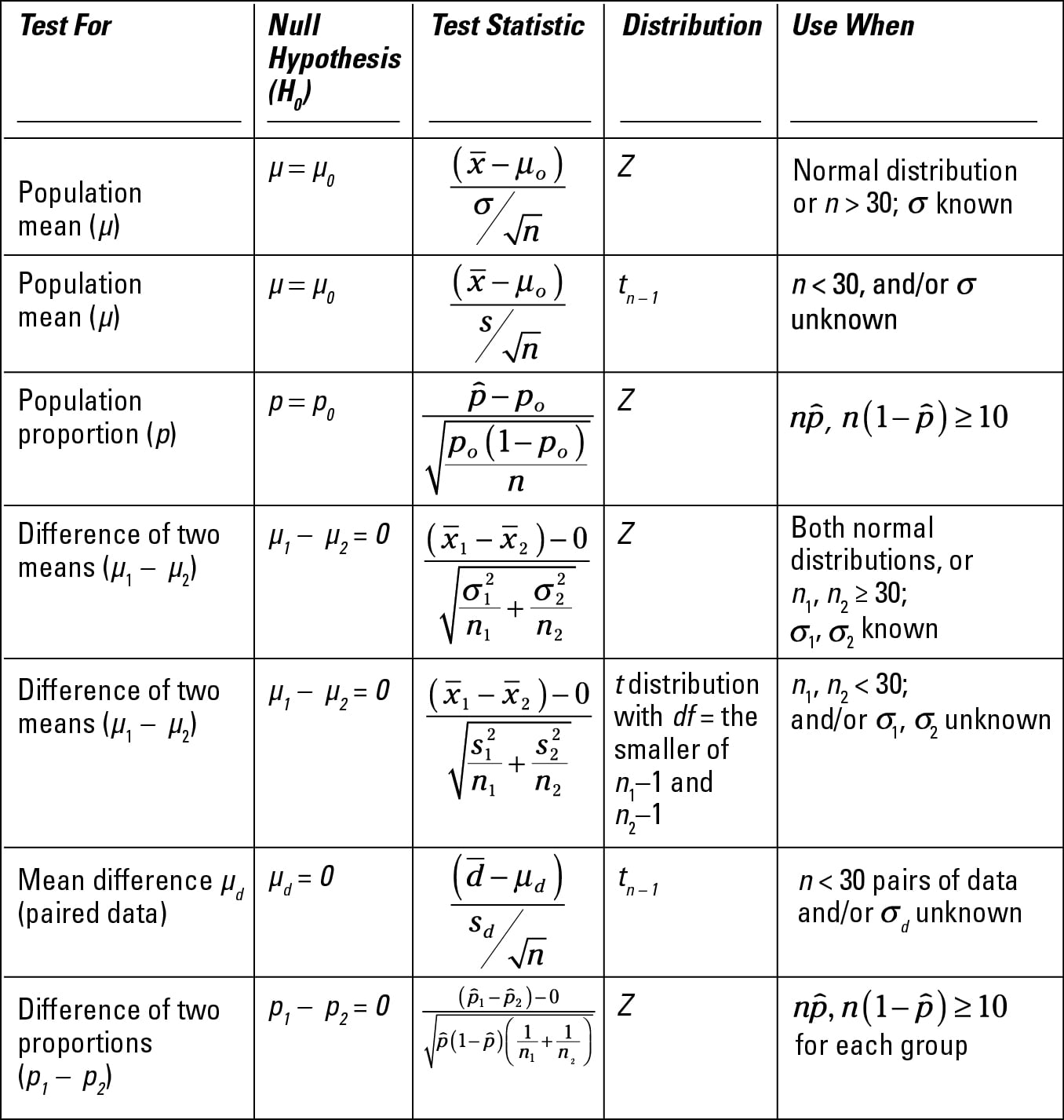##### Statistics For Dummies, 2nd EditionWhether you’re studying for an exam or just want to make sense of data around you every day, knowing how and when to use data analysis techniques and formulas of statistics will help.

Being able to make the connections between those statistical techniques and formulas is perhaps even more important. It builds confidence when tackling statistical problems and solidifies your strategies for completing statistical projects.

## Understanding formulas for common statistics

After data has been collected, the first step in analyzing it is to crunch out some descriptive statistics to get a feeling for the data. For example:

• Where is the center of the data located?

• How spread out is the data?

• How correlated are the data from two variables?

The most common descriptive statistics are in the following table, along with their formulas and a short description of what each one measures.## Statistically figuring sample size

When designing a study, the sample size is an important consideration because the larger the sample size, the more data you have, and the more precise your results will be (assuming high-quality data). If you know the level of precision you want (that is, your desired margin of error), you can calculate the sample size needed to achieve it.

To find the sample size needed to estimate a population mean (µ), use the following formula:In this formula, MOE represents the desired margin of error (which you set ahead of time), and σ represents the population standard deviation. If σ is unknown, you can estimate it with the sample standard deviation, s, from a pilot study; z* is the critical value for the confidence level you need.

## Surveying statistical confidence intervals

In statistics, a confidence interval is an educated guess about some characteristic of the population. A confidence interval contains an initial estimate plus or minus a margin of error (the amount by which you expect your results to vary, if a different sample were taken).

The following table shows formulas for the components of the most common confidence intervals and keys for when to use them.## Checking out statistical confidence interval critical values

Critical values (z*-values) are an important component of confidence intervals (the statistical technique for estimating population parameters).

The z*-value, which appears in the margin of error formula, measures the number of standard errors to be added and subtracted in order to achieve your desired confidence level (the percentage confidence you want). The following table shows common confidence levels and their corresponding z*-values.

Confidence Level z*– value
80% 1.28
85% 1.44
90% 1.64
95% 1.96
98% 2.33
99% 2.58

## Handling statistical hypothesis tests

You use hypothesis tests to challenge whether some claim about a population is true (for example, a claim that 40 percent of Americans own a cellphone).

To test a statistical hypothesis, you take a sample, collect data, form a statistic, standardize it to form a test statistic (so it can be interpreted on a standard scale), and decide whether the test statistic refutes the claim. The following table lays out the important details for hypothesis tests.